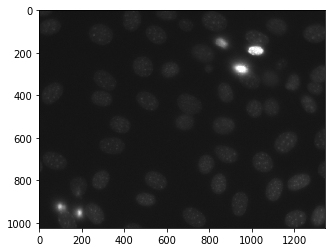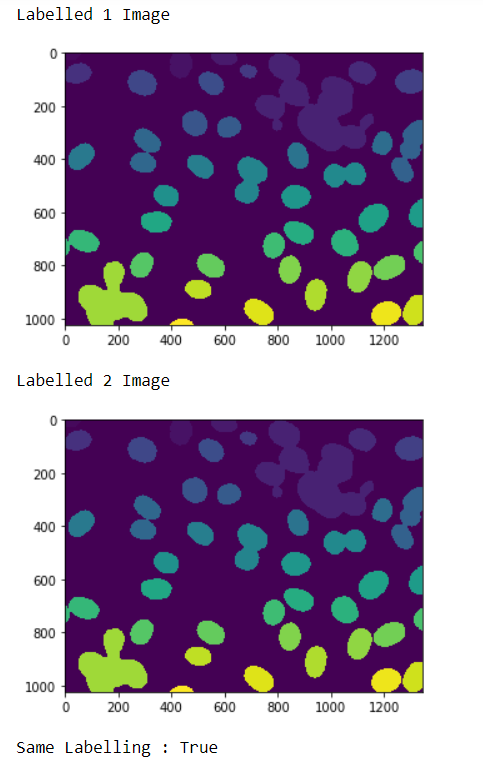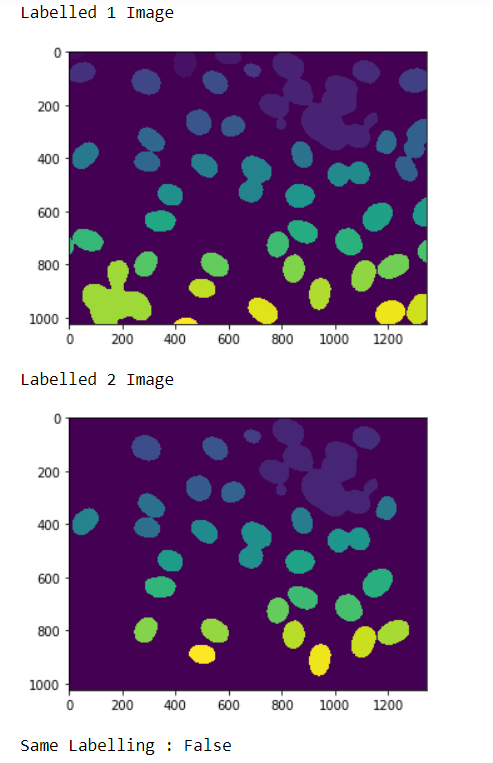Skip to content
Related Articles
Mahotas – Checking if two images represent same labeling
• Last Updated : 17 May, 2021

In this article we will see how we can check if the two images represent the same labeling in mahotas. For this we are going to use the fluorescent microscopy image from a nuclear segmentation benchmark. We can get the image with the help of command given below

`mahotas.demos.nuclear_image()`

Below is the nuclear_imageIn order to do this we will use mahotas.labeled.is_same_labeling method

Syntax : mahotas.labeled.is_same_labeling(label1. labeled2)
Argument : It takes two labelled image as argument
Return : It returns bool

Note : The input of the this should should be the filtered image object which is labeled
In order to filter the image we will take the image object which is numpy.ndarray and filter it with the help of indexing, below is the command to do this

`image = image[:, :, 0]`

Example 1 :

## Python3

 `# importing required libraries``import` `mahotas``import` `numpy as np``from` `pylab ``import` `imshow, show``import` `os` `# loading nuclear image``f1 ``=` `mahotas.demos.load(``'nuclear'``)` `# setting filter to the image``f1 ``=` `f1[:, :, ``0``]`  `# setting gaussian filter``f1 ``=` `mahotas.gaussian_filter(f1, ``4``)` `# setting threshold value``f1 ``=` `(f1> f1.mean())` `# creating a labeled image``labeled1, n_nucleus1 ``=` `mahotas.label(f1)` `# showing the labeleed image``print``(``"Labelled 1 Image"``)``imshow(labeled1)``show()` `# loading nuclear image``f2 ``=` `mahotas.demos.load(``'nuclear'``)` `# setting filter to the image``f2 ``=` `f2[:, :, ``0``]` `# setting gaussian filter``f2 ``=` `mahotas.gaussian_filter(f2, ``4``)` `# setting threshold value``f2 ``=` `(f2> f2.mean())` `# creating a labeled image``labeled2, n_nucleus2 ``=` `mahotas.label(f2)`  `# showing the labeleed image``print``(``"Labelled 2 Image"``)``imshow(labeled2)``show()` `# c hecking if both the labeled images are same``check ``=` `mahotas.labeled.is_same_labeling(labeled1, labeled2)` `# printing check``print``(``"Same Labelling : "``+` `str``(check))`

Output :`Same Labelling : True`

Example 2 :

## Python3

 `# importing required libraries``import` `mahotas``import` `numpy as np``from` `pylab ``import` `imshow, show``import` `os` `# loading nuclear image``f1 ``=` `mahotas.demos.load(``'nuclear'``)` `# setting filter to the image``f1 ``=` `f1[:, :, ``0``]`  `# setting gaussian filter``f1 ``=` `mahotas.gaussian_filter(f1, ``4``)` `# setting threshold value``f1 ``=` `(f1> f1.mean())` `# creating a labeled image``labeled1, n_nucleus1 ``=` `mahotas.label(f1)` `# showing the labeleed image``print``(``"Labelled 1 Image"``)``imshow(labeled1)``show()` `# loading nuclear image``f2 ``=` `mahotas.demos.load(``'nuclear'``)` `# setting filter to the image``f2 ``=` `f2[:, :, ``0``]` `# setting gaussian filter``f2 ``=` `mahotas.gaussian_filter(f2, ``4``)` `# setting threshold value``f2 ``=` `(f2> f2.mean())` `# creating a labeled image``labeled2, n_nucleus2 ``=` `mahotas.label(f2)` `# removing border``labeled2 ``=` `mahotas.labeled.remove_bordering(labeled2)`  `# showing the labeleed image``print``(``"Labelled 2 Image"``)``imshow(labeled2)``show()` `# checking if both the labeled images are same``check ``=` `mahotas.labeled.is_same_labeling(labeled1, labeled2)` `# printing check``print``(``"Same Labelling : "``+` `str``(check))`

Output :`Same Labelling : False`

Attention geek! Strengthen your foundations with the Python Programming Foundation Course and learn the basics.

To begin with, your interview preparations Enhance your Data Structures concepts with the Python DS Course. And to begin with your Machine Learning Journey, join the Machine Learning – Basic Level Course

My Personal Notes arrow_drop_up